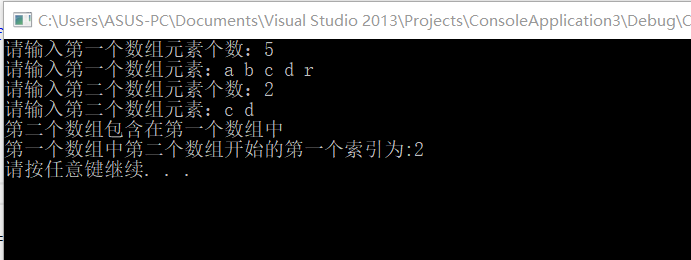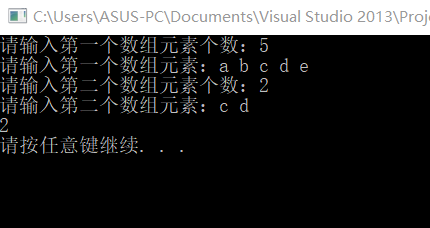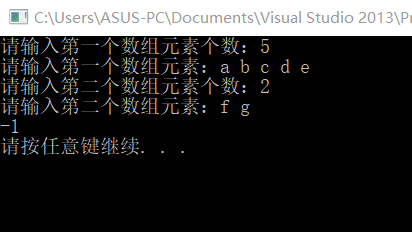2021-06-11 10:55

# 关于c++的题目，菜鸟求解答

• 点赞
• 写回答
• 关注问题
• 收藏
• 邀请回答

#### 6条回答默认 最新

•登楼流樱 2021-06-11 14:20
已采纳
``````#include <iostream>
#include <string>

using namespace std;

int main()
{
char a,b;
int numA, numB;
cout << "请输入第一个数组元素个数：";
cin >> numA;
cout << "请输入第一个数组元素：";
for (int i = 0; i < numA; ++i)
cin >> a[i];
cin.clear();
cin.sync();
cout << "请输入第二个数组元素个数：";
cin >> numB;
cout << "请输入第二个数组元素：";
for (int i = 0; i < numB; ++i)
cin >> b[i];
int num = 0;		//第二个数组包含在第一个数组的个数
string index;		//存放第二个数组在第一个数组的索引
for (int j = 0; j < numB; j++)		//第二个数组的元素与第一数组的元素遍历
{
for (int k = 0; k < numA; k++)
{
if (b[j] == a[k])
{
index += to_string(k);
num++;
break;
}
}
}

if (num == numB)
{
cout << "第二个数组包含在第一个数组中" << endl;
cout << "第一个数组中第二个数组开始的第一个索引为:" << index.substr(0,1) << endl;
}
else
cout << "第二个数组不被包含在第一个数组";

system("pause");
return 0;
}``````点赞 1 打赏 评论
•双重遍历检索就可以了

``````#include <iostream>
using namespace std;
void main()
{
char a, b;
int n, m, i, j, k, p;
cout << "请分别输入两个数组的长度:";
cin >> n >> m;
getchar();
cout << "请输入第一个数组的内容:";
for (i = 0; i<n; i++)
cin >> a[i];
getchar();
cout << "请输入第二个数组的内容:";
for (i = 0; i<m; i++)
cin >> b[i];
//
p = -1;
for (i = 0; i<n; i++)
{
k = i;
for (j = 0; j<m; j++)
{
if (b[j] != a[k++])
break;
}
if (j == m)
{
p = i;
break;
}
}
if (p == -1)
cout << "第一个数组不包含第二个数组" << endl;
else
cout << "第一个数组包含第二个数组，起点为：" << p << endl;
}``````
点赞 打赏 评论
•登楼流樱 2021-06-11 15:08
``````#include <iostream>
#include <string>

using namespace std;

int test(char a[], char b[], int numA, int numB)
{
int num = 0;		//第二个数组包含在第一个数组的个数
string index;		//存放第二个数组在第一个数组的索引
for (int j = 0; j < numB; j++)		//第二个数组的元素与第一数组的元素遍历
{
for (int k = 0; k < numA; k++)
{
if (b[j] == a[k])
{
index += to_string(k);
num++;
break;
}
}
}
if (num == numB)
{
return stoi(index.substr(0, 1));
}
else
return -1;
}

int main()
{
char a,b;
int numA, numB;
cout << "请输入第一个数组元素个数：";
cin >> numA;
cout << "请输入第一个数组元素：";
for (int i = 0; i < numA; ++i)
cin>>a[i];
cin.clear();
cin.sync();
cout << "请输入第二个数组元素个数：";
cin >> numB;
cout << "请输入第二个数组元素：";
for (int i = 0; i < numB; ++i)
cin >> b[i];
cout << test(a, b, numA, numB) << endl;
system("pause");
return 0;
}
``````点赞 打赏 评论
•路途遥远gg 2021-06-11 17:20

是不是想这样用string来实现？ 非常香

int a1gaoshanwdnmd(const char* param1,const char* param2)

{
std::string strA;

std::string strB;

int ret = -1;

if(strA.find(strB) != strA.nops)

{
ret = 1;

}

return ret;

}

点赞 打赏 评论
•有问必答小助手 2021-06-15 18:35

您好，我是有问必答小助手，您的问题已经有小伙伴解答了，您看下是否解决，可以追评进行沟通哦~

如果有您比较满意的答案 / 帮您提供解决思路的答案，可以点击【采纳】按钮，给回答的小伙伴一些鼓励哦～～